Next: The power spectrum and Up: Fourier analysis: The sine Previous: Fourier analysis: The sine

Fourier transforms

Transformations which take functions, e.g. x, y as arguments and return functions as results are called operators. The direct and inverse Fourier transform,, and the convolution, *, are operators defined in the following way: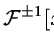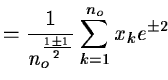(12.2) [x*y](l)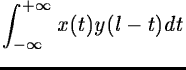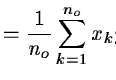(12.3)

where square brackets, [ ], indicate the order of the operators and round brackets, (), indicate the arguments of the input and output functions. Without loss of generality we consider here functions with zero mean value. Note that because of the finite and infinite correlation length of stochastic and periodic series, respectively, no unique normalization C applies in the continuous case.

The discrete operators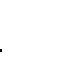and * are well defined only for observations and frequencies which are spaced evenly by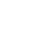and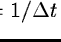, respectively, and span ranges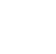and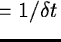. Then and only thenreduces to orthogonal matrices. It follows directly from Eq. (12.2) that we implicitly assume that the observations and their transforms are periodic with the periodsand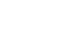, respectively. The assumption is of consequence only for data strings which are short compared to the investigated periods or coherence lengths or for a sampling which is coarse compared to these two quantities. Such situations should be avoided also in the general case of unevenly sampled observations.

The following properties ofand * are noteworthy: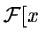=(12.4)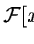=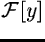(12.5)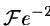=(12.6)

where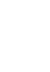denotes the Dirac symbol: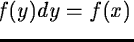. In the discrete case,assumes the value no for x and 0elsewhere.Next: The power spectrum and Up: Fourier analysis: The sine Previous: Fourier analysis: The sine
Petra Nass
1999-06-15